## Solving SBT lesson 1 Rectangle, cube (C3 Math 7 – Horizon) – Math book

Solve SBT lesson 1 Rectangular box, cube (C3 Math 7 – Horizon)
============

### Solve problem 1 page 53 SBT Math 7 Creative horizon episode 1 – CTST

Let ABCD.EFGH rectangle, know side AB = 5 cm, BC = 4 cm, AE = 3 cm.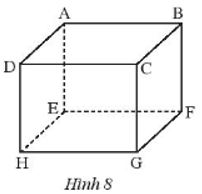a) Give the lengths of the remaining sides.

b) Name and draw diagonal lines.

c) State the angles of the vertices F, C, and D.

## Detailed instructions for solving Lesson 1

Solution method

We rely on the property that the faces of the rectangular box are all rectangles from which to infer pairs of equal sides, determine the diagonal in the figure by defining the diagonal in the rectangular box and from the faces of the box. State the angles corresponding to the vertices.

Detailed explanation

a) Since ABCD.EFGH is a rectangular box, ABCD, AEHD, BCGF, ABFE, ACGH, EFGH are rectangles.

So we have: CD = HG = EF = AB = 5 cm; AD = HE = GF = BC = 4 cm;

DH = CG = BF = AE = 3 cm.

b) The diagonals of the rectangular box ABCD.EFGH are AG, DF, EC, BH, we draw as shown below:

c) The vertex angles F are: angle BFE, angle BFG, angle EFG.

The vertex angles C are: Angle BCD, Angle DCG, Angle BCG.

–>

— *****

### Solve problem 2 page 53 SBT Math 7 Creative horizon episode 1 – CTST

Given cube ABCD.MNPQ.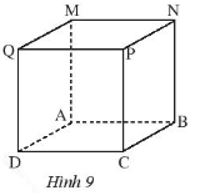a) Given BC = 4 cm, calculate the remaining sides.

b) Name the faces of the cube.

c) Draw diagonal lines starting from vertices P, Q.

d) State the vertices B and P of the cube.

## Detailed instructions for solving Lesson 2

Solution method

Use properties of cubes.

Detailed explanation

a) Since ABCD.MNPQ is a cube, it has 12 equal sides.

Which BC = 4 cm.

So AB = AD = CD = BN = AM = DQ = PC = QM = MN = NP = PQ = 4 cm.

b) The faces of the above cube are: face ABCD, face ABNM, face BCPN, face CDQP, face DAQM, face MNPQ.

c) The diagonal from the vertex P is PA, the diagonal from the vertex Q is QB.

d) The angles of vertex B are: angle ABC, angle ABN, angle NBC.

The vertex angles P are: Angle QPN, Angle QPC, Angle CPN

–>

— *****

### Solution 3 page 53 SBT Math 7 Creative horizon episode 1 – CTST

Find objects and structures in life that have the shape of a rectangular box, a cube.

## Detailed instructions for solving Lesson 3

Solution method

Observe surrounding objects and structures.

Detailed explanation

– Example of a rectangular box: fish tank, cake box, ….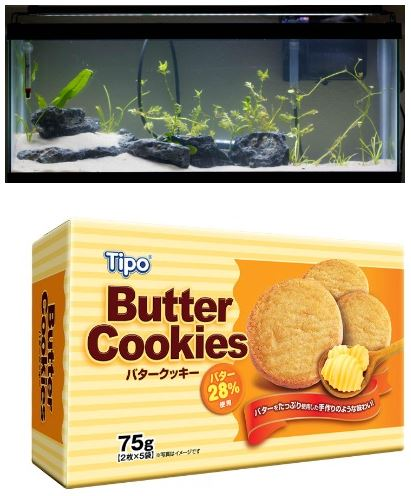– Example of a cube: dice, rubik’s cube, etc.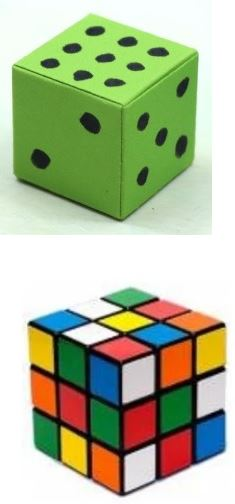–>

— *****

### Solution 4 page 53 SBT Math 7 Creative horizon episode 1 – CTST

Draw more edges to get a rectangular box, a cube.## Detailed instructions for solving Lesson 4

Solution method

We rely on how to draw dashed and solid lines to complete the missing rectangles and cubes.

Detailed explanation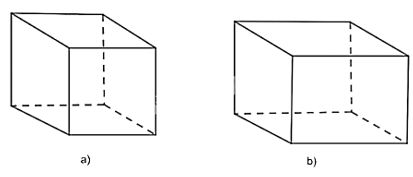–>

— *****

### Solve problem 5 page 53 SBT Math 7 Creative horizon episode 1 – CTST

Which of the following two cards can be folded into a rectangular box?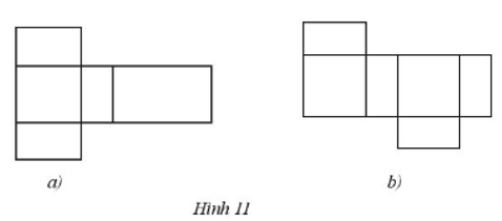## Detailed instructions for solving Lesson 5

Solution method

We see that a rectangular box is made up of rectangles, but each pair of opposite faces will be equal

Detailed explanation

Of the two cards given above, the one in Figure 11b) folds into a rectangular box.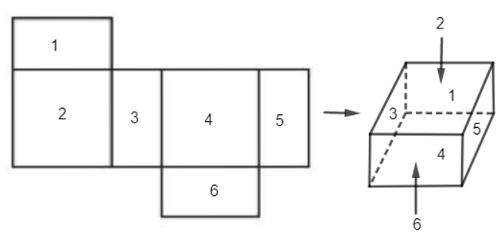–>

— *****

### Solution 6 page 54 SBT Math 7 Creative horizon episode 1 – CTST

Indicate which pair of sides fold together to form a cube.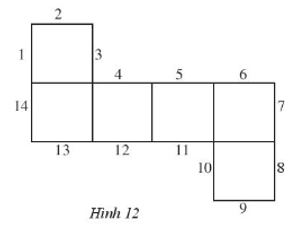## Detailed instructions for solving Lesson 6

Solution method

We can draw a cube and then denote the pairs of opposite sides from which we will find a pair of folded edges to form a cube.

Detailed explanation

Each of the following pairs of numbers is an edge symbol that joins together to get a cube:

3 and 4; 5 and 2; 6 and 1; 7 and 14; 8 and 13; 9 and 12; 10 and 11

–>

— *****